## 2013-09-19

### SSRS - Line Chart - Create stacked line and start from 0

Stakeholders want a Stacked Line Chart to illustrate the difference of accumulative value between Actual and Forecast, unfortunately, SSRS doesn't provide this type of chart. There are 5 columns in original dataset: ProjectID, Project_Name, Category(Actual or Forecast), Transaction_Period, and Amount.

First of all, I need to check initial result of graph from original dataset, so I drag a Line Chart in the report, in Values section, add field Amount; in Category Groups, add field Transaction_Period; in Series Groups, add field Category.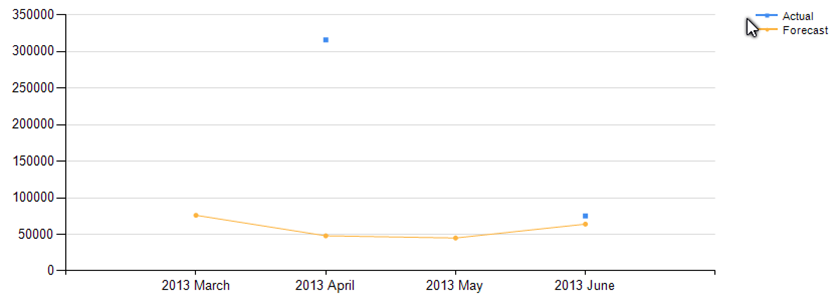As you can see from the chart above, it is a Line with Markers Chart, if I use Line Chart, then I couldn't see any dots of Actual because the group doesn't have sequential data.

For meeting the requirement, there are some challenges I have to work out:

1. Build a line to connect each marker.
2. Lines start from 0.
3. Calculate accumulative value for each group.

Let's take a look at the evolution of the chart:

1. For challenge 1, add virtual transaction period to build sequential data for each group.
2. For challenge 2, add extra extra virtual start and finish period for each project.
3. For challenge 3, add new column in original dataset called Amount_Accumulate, later I will explain the logic and how to realize it in the query.

Please see the result of stage 1: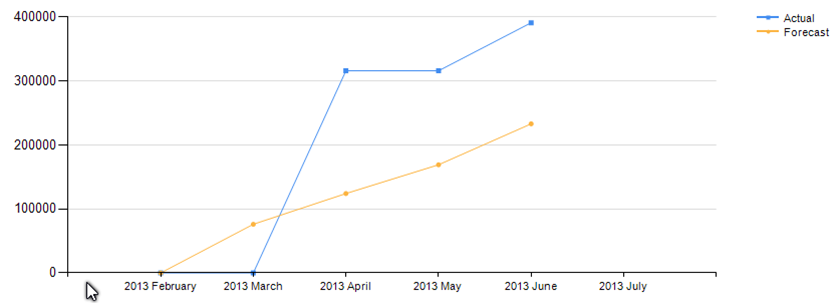Note: If you can't see all labels displayed in horizontal axis, right-click the axis, select Horizontal Axis Properties, under Axis Options group, in Axis range and interval section, in Interval field, change default value from Auto to 1.

Right-click horizontal axis, select Horizontal Axis Properties, under Axis Options group, in Axis range and interval section, in Side margins field, change default value from Auto to Disabled, switch to Labels group, tick Hide first and last labels along this axis. Please see the result of stage 2: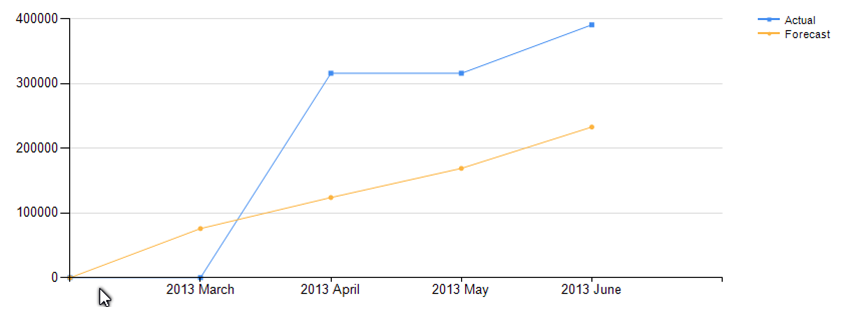For the line between virtual start period(2013 February) and 2013 March, it is overlapped with horizontal axis because both of them along with 0 value. I start to think how to straighten the line, my logic is to distribute the value among virtual periods. The logic will be explained in the query, and please see the result of stage 3: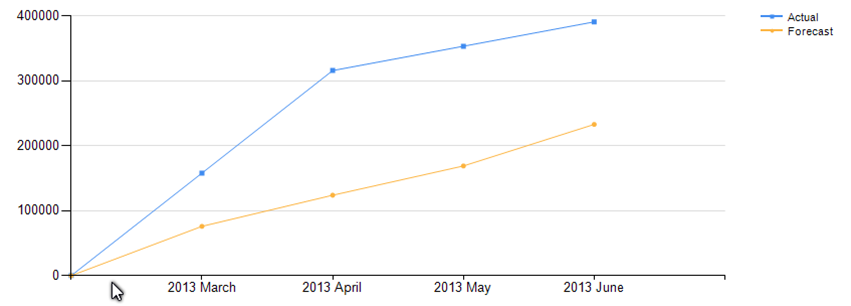The last step is to hide marker of virtual periods in the chart, right-click the line, select Series Properties, under Markers group, in Marker type field, set expression for Marker Type:
=IIF(Fields!Amount.Value > 0, "Square", "None")
Please see the final result: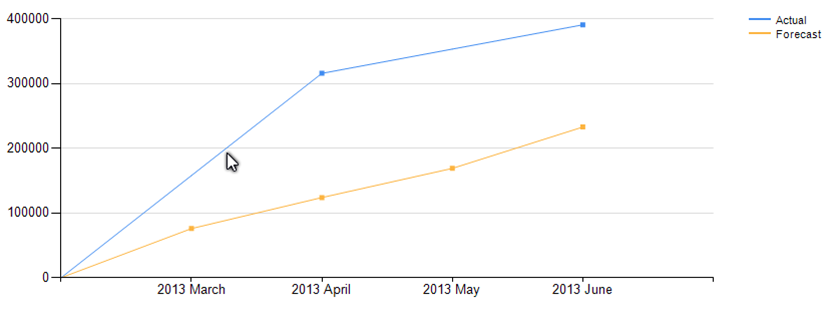Please see the following explanation of the logic in the query:
SET NOCOUNT ON;

--For debugging
--/*
DECLARE @ProjectIDs        VARCHAR(500)

SET @ProjectIDs = '2126, 2125, 2133, 2179, 2198, 2214, 2218, 2257, 2258'
--*/

DECLARE @Chart_Original TABLE
(
ProjectID                     VARCHAR(50),
Project_Name            VARCHAR(500),
Transaction_Period   VARCHAR(50),
Transaction_Year        INT,
Transaction_Month     INT,
Amount                        FLOAT,
Category                     VARCHAR(50),
Amount_Accumulate  FLOAT
)

DECLARE @Chart_Final TABLE
(
ProjectID                    VARCHAR(50),
Project_Name           VARCHAR(500),
Transaction_Period  VARCHAR(50),
Transaction_Year       INT,
Transaction_Month    INT,
Amount                       FLOAT,
Category                    VARCHAR(50),
Amount_Accumulate FLOAT
)

DECLARE @ProjectID                          VARCHAR(50),
@ProjectID_Previous                     VARCHAR(50),
@Project_Name                              VARCHAR(500),
@Transaction_Period                     VARCHAR(50),
@Transaction_Period_Previous    VARCHAR(50),
@Transaction_Period_tmp            DATE,
@Transaction_Year                         INT,
@Transaction_Month                      INT,
@Amount                                         FLOAT,
@Amount_Accumulate                   FLOAT,
@Category                                      VARCHAR(50),
@Category_Previous                     VARCHAR(50),
@Counts_MissedPeriod                INT,
@Count                                             INT

SET @ProjectID_Previous = ''
SET @Category_Previous = ''
SET @Amount_Accumulate = 0
SET @Counts_MissedPeriod = 0
SET @Count = 1

--==============================================================
INSERT INTO @Chart_Original
SELECT    ProjectID = Parent_ProjectID
, Project_Name = Parent_ProjectName
, Transaction_Period = CONVERT(VARCHAR, YEAR(TransDate)) + ' ' + DATENAME(M, TransDate)
, Transaction_Year = YEAR(TransDate)
, Transaction_Month = MONTH(TransDate)
, Amount = SUM(Amount)
, Category
, NULL
FROM        AX_InvoiceForecast
WHERE    Parent_ProjectID COLLATE SQL_Latin1_General_CP1_CI_AS IN (SELECT * FROM PortfolioDW.dbo.udfSplitStringArray(@ProjectIDs))
GROUP BY
Parent_ProjectID
, Parent_ProjectName
, CONVERT(VARCHAR, YEAR(TransDate)) + ' ' + DATENAME(M, TransDate)
, YEAR(TransDate)
, MONTH(TransDate)
, Category

--SELECT * FROM @Chart_Original

--Add extra period with 0 value before starting period and extra period with NULL after last period for each project
DECLARE cur_projects CURSOR FOR SELECT ProjectID, Project_Name FROM @Chart_Original GROUP BY ProjectID, Project_Name
OPEN cur_projects
FETCH NEXT FROM cur_projects INTO @ProjectID, @Project_Name

WHILE @@FETCH_STATUS = 0
BEGIN
--For virtual start period
SET @Transaction_Period_tmp =    (
SELECT    TOP 1 Transaction_Period
FROM       @Chart_Original
WHERE    ProjectID = @ProjectID
ORDER BY Transaction_Year, Transaction_Month
)

SET @Transaction_Period_tmp = DATEADD(M, -1, CONVERT(DATE, @Transaction_Period_tmp))

INSERT INTO @Chart_Final
VALUES    (
@ProjectID,
@Project_Name,
CONVERT(VARCHAR, YEAR(@Transaction_Period_tmp)) + ' ' + DATENAME(M, @Transaction_Period_tmp),
YEAR(@Transaction_Period_tmp),
MONTH(@Transaction_Period_tmp),
0,
'Actual',
0
),
(
@ProjectID,
@Project_Name,
CONVERT(VARCHAR, YEAR(@Transaction_Period_tmp)) + ' ' + DATENAME(M, @Transaction_Period_tmp),
YEAR(@Transaction_Period_tmp),
MONTH(@Transaction_Period_tmp),
0,
'Forecast',
0
)
--==================================================
--For virtual finish period
SET @Transaction_Period_tmp =    (
SELECT    TOP 1 Transaction_Period
FROM       @Chart_Original
WHERE    ProjectID = @ProjectID
ORDER BY Transaction_Year DESC, Transaction_Month DESC
)

SET @Transaction_Period_tmp = DATEADD(M, 1, CONVERT(DATE, @Transaction_Period_tmp))

INSERT INTO @Chart_Final
VALUES    (
@ProjectID,
@Project_Name,
CONVERT(VARCHAR, YEAR(@Transaction_Period_tmp)) + ' ' + DATENAME(M, @Transaction_Period_tmp),
YEAR(@Transaction_Period_tmp),
MONTH(@Transaction_Period_tmp),
NULL,
'Forecast',
NULL
)

FETCH NEXT FROM cur_projects INTO @ProjectID, @Project_Name
END
CLOSE cur_projects
DEALLOCATE cur_projects

INSERT INTO @Chart_Final
SELECT * FROM @Chart_Original

--SELECT * FROM @Chart_Final ORDER BY ProjectID, Category, Transaction_Year, Transaction_Month

DECLARE cur_projects CURSOR FOR SELECT   ProjectID,
Project_Name,
Transaction_Period,
Transaction_Year,
Transaction_Month,
Amount,
Category
FROM    @Chart_Final
ORDER BY
ProjectID
, Category
, Transaction_Year
, Transaction_Month
OPEN cur_projects
FETCH NEXT FROM cur_projects INTO     @ProjectID,
@Project_Name,
@Transaction_Period,
@Transaction_Year,
@Transaction_Month,
@Amount,
@Category

WHILE @@FETCH_STATUS = 0
BEGIN
IF @ProjectID_Previous <> @ProjectID OR @Category_Previous <> @Category
SET @Amount_Accumulate = 0

IF @ProjectID_Previous = @ProjectID
BEGIN
SET @Counts_MissedPeriod = 0
SET @Count = 1
SET @Transaction_Period_tmp = DATEADD(M, 1, CONVERT(DATE, @Transaction_Period_Previous))

--For building line to connect markers in Line Chart, insert any missed period between existing period
WHILE CONVERT(DATE, @Transaction_Period_tmp) < CONVERT(DATE, @Transaction_Period)
BEGIN
INSERT INTO @Chart_Final
VALUES    (
@ProjectID,
@Project_Name,
CONVERT(VARCHAR, YEAR(@Transaction_Period_tmp)) + ' ' + DATENAME(M, @Transaction_Period_tmp),
YEAR(@Transaction_Period_tmp),
MONTH(@Transaction_Period_tmp),
0,
@Category,
@Amount_Accumulate
)

SET @Transaction_Period_tmp = DATEADD(M, 1, CONVERT(DATE, @Transaction_Period_tmp))
SET @Transaction_Period_Previous = CONVERT(VARCHAR, YEAR(@Transaction_Period_tmp)) + ' ' + DATENAME(M, @Transaction_Period_tmp)
SET @Counts_MissedPeriod = @Counts_MissedPeriod + 1
END

--For displaying stacked line in Line Chart by calculating accumulative amount for each period
SET @Amount_Accumulate = @Amount_Accumulate + @Amount

UPDATE    @Chart_Final
SET        Amount_Accumulate = @Amount_Accumulate
WHERE    ProjectID = @ProjectID
AND Transaction_Period = @Transaction_Period
AND Category = @Category

--For displaying straight line in Line Chart, distribute value into missed period
WHILE @Count <= @Counts_MissedPeriod
BEGIN
--SELECT @Amount, @Counts_MissedPeriod, @Count
--SELECT ISNULL(@Amount, 0)/1.0/(@Counts_MissedPeriod+1)*(@Counts_MissedPeriod-@Count+1)
SET @Transaction_Period_tmp = DATEADD(M, -@Count, CONVERT(DATE, @Transaction_Period))
--SELECT @Transaction_Period_tmp
UPDATE    @Chart_Final
SET        Amount_Accumulate = Amount_Accumulate + ISNULL(@Amount, 0)/1.0/(@Counts_MissedPeriod+1)*(@Counts_MissedPeriod-@Count+1)
WHERE    ProjectID = @ProjectID
AND Transaction_Period = CONVERT(VARCHAR, YEAR(@Transaction_Period_tmp)) + ' ' + DATENAME(M, @Transaction_Period_tmp)
AND Category = @Category
AND Amount = 0

SET @Count = @Count + 1
END
END

SET    @ProjectID_Previous = @ProjectID
SET    @Transaction_Period_Previous = @Transaction_Period
SET    @Category_Previous = @Category

FETCH NEXT FROM cur_projects INTO    @ProjectID,
@Project_Name,
@Transaction_Period,
@Transaction_Year,
@Transaction_Month,
@Amount,
@Category
END
CLOSE cur_projects
DEALLOCATE cur_projects
--==============================================================
SELECT    *
FROM    @Chart_Final
ORDER BY
ProjectID
, Project_Name
, Category
, Transaction_Year
, Transaction_Month

The @Chart_Final table is used to visualize result of graph, if stakeholders want to know actual comparison information, I would suggest using original dataset @Chart_Original to prevent pulling down aggregation value of dataset's population.